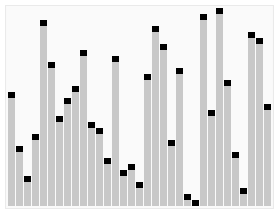# php基础算法有哪几种`\$arr=array(11,3,56,62,21,66,32,78,36,76,39,88,34);`

### 1.冒泡排序

1.比较相邻的元素。如果第一个比第二个大，就交换他们两个。

2.对每一对相邻元素作同样的工作，从开始第一对到结尾的最后一对。在这一点，最后的元素应该会是最大的数。

3.针对所有的元素重复以上的步骤，除了最后一个。

4.持续每次对越来越少的元素重复上面的步骤，直到没有任何一对数字需要比较。

```\$arr=array(1,43,54,62,21,66,32,78,36,76,39);
function bubbleSort (\$arr)
{
\$len = count(\$arr);
//该层循环控制 需要冒泡的轮数
for (\$i=1; \$i<\$len; \$i++) {
//该层循环用来控制每轮 冒出一个数 需要比较的次数
for (\$k=0; \$k<\$len-\$i; \$k++) {
if(\$arr[\$k] > \$arr[\$k+1]) {
\$tmp = \$arr[\$k+1]; // 声明一个临时变量
\$arr[\$k+1] = \$arr[\$k];
\$arr[\$k] = \$tmp;
}
}
}
return \$arr;
}```### 2.选择排序

```//实现思路 双重循环完成，外层控制轮数，当前的最小值。内层 控制的比较次数
function select_sort(\$arr) {
//\$i 当前最小值的位置， 需要参与比较的元素
for(\$i=0, \$len=count(\$arr); \$i<\$len-1; \$i++) {
//先假设最小的值的位置
\$p = \$i;
//\$j 当前都需要和哪些元素比较，\$i 后边的。
for(\$j=\$i+1; \$j<\$len; \$j++) {
//\$arr[\$p] 是 当前已知的最小值
if(\$arr[\$p] > \$arr[\$j]) {
//比较，发现更小的,记录下最小值的位置；并且在下次比较时，应该采用已知的最小值进行比较。
\$p = \$j;
}
}
//已经确定了当前的最小值的位置，保存到\$p中。
//如果发现 最小值的位置与当前假设的位置\$i不同，则位置互换即可
if(\$p != \$i) {
\$tmp = \$arr[\$p];
\$arr[\$p] = \$arr[\$i];
\$arr[\$i] = \$tmp;
}
}
//返回最终结果
return \$arr;
}```### 3.插入排序

1.从第一个元素开始，该元素可以认为已经被排序

2.取出下一个元素，在已经排序的元素序列中从后向前扫描

3.如果该元素（已排序）大于新元素，将该元素移到下一位置

4.重复步骤3，直到找到已排序的元素小于或者等于新元素的位置

5.将新元素插入到该位置中

6.重复步骤2

```function insert_sort(\$arr)
{
\$len=count(\$arr);
for(\$i=1; \$i<\$len; \$i++) {
//获得当前需要比较的元素值。
\$tmp = \$arr[\$i];
//内层循环控制 比较 并 插入
for(\$j=\$i-1; \$j>=0; \$j--) {
//\$arr[\$i];//需要插入的元素; \$arr[\$j];//需要比较的元素
if(\$tmp < \$arr[\$j]) {
//发现插入的元素要小，交换位置
//将后边的元素与前面的元素互换
\$arr[\$j+1] = \$arr[\$j];
//将前面的数设置为 当前需要交换的数
\$arr[\$j] = \$tmp;
} else {
//如果碰到不需要移动的元素
//由于是已经排序好是数组，则前面的就不需要再次比较了。
break;
}
}
}
//将这个元素 插入到已经排序好的序列内。
//返回
return \$arr;
}```### 4.快速排序

1.从数列中挑出一个元素，称为 “基准”（pivot），

2.重新排序数列，所有元素比基准值小的摆放在基准前面，所有元素比基准值大的摆在基准的后面（相同的数可以到任一边）。在这个分区退出之后，该基准就处于数列的中间位置。这个称为分区（partition）操作。

3.递归地（recursive）把小于基准值元素的子数列和大于基准值元素的子数列排序。

```function quick_sort(\$arr)
{
//判断参数是否是一个数组
if(!is_array(\$arr)) return false;
//递归出口:数组长度为1，直接返回数组
\$length = count(\$arr);
if(\$length<=1) return \$arr;
//数组元素有多个,则定义两个空数组
\$left = \$right = array();
//使用for循环进行遍历，把第一个元素当做比较的对象
for(\$i=1; \$i<\$length; \$i++)
{
//判断当前元素的大小
if(\$arr[\$i]<\$arr){
\$left[]=\$arr[\$i];
}else{
\$right[]=\$arr[\$i];
}
}
//递归调用
\$left=quick_sort(\$left);
\$right=quick_sort(\$right);
//将所有的结果合并
return array_merge(\$left,array(\$arr),\$right);
}```• 相关标签：php算法
• 相关文章

相关视频